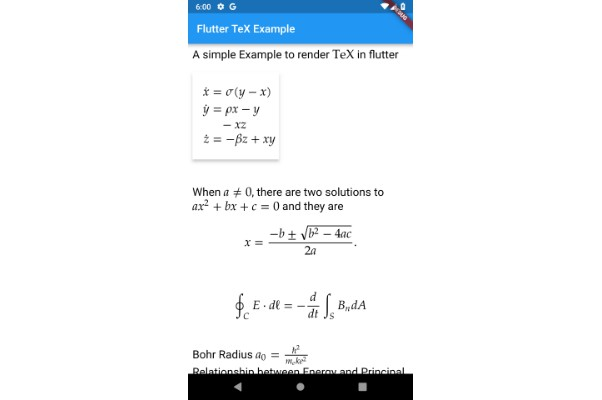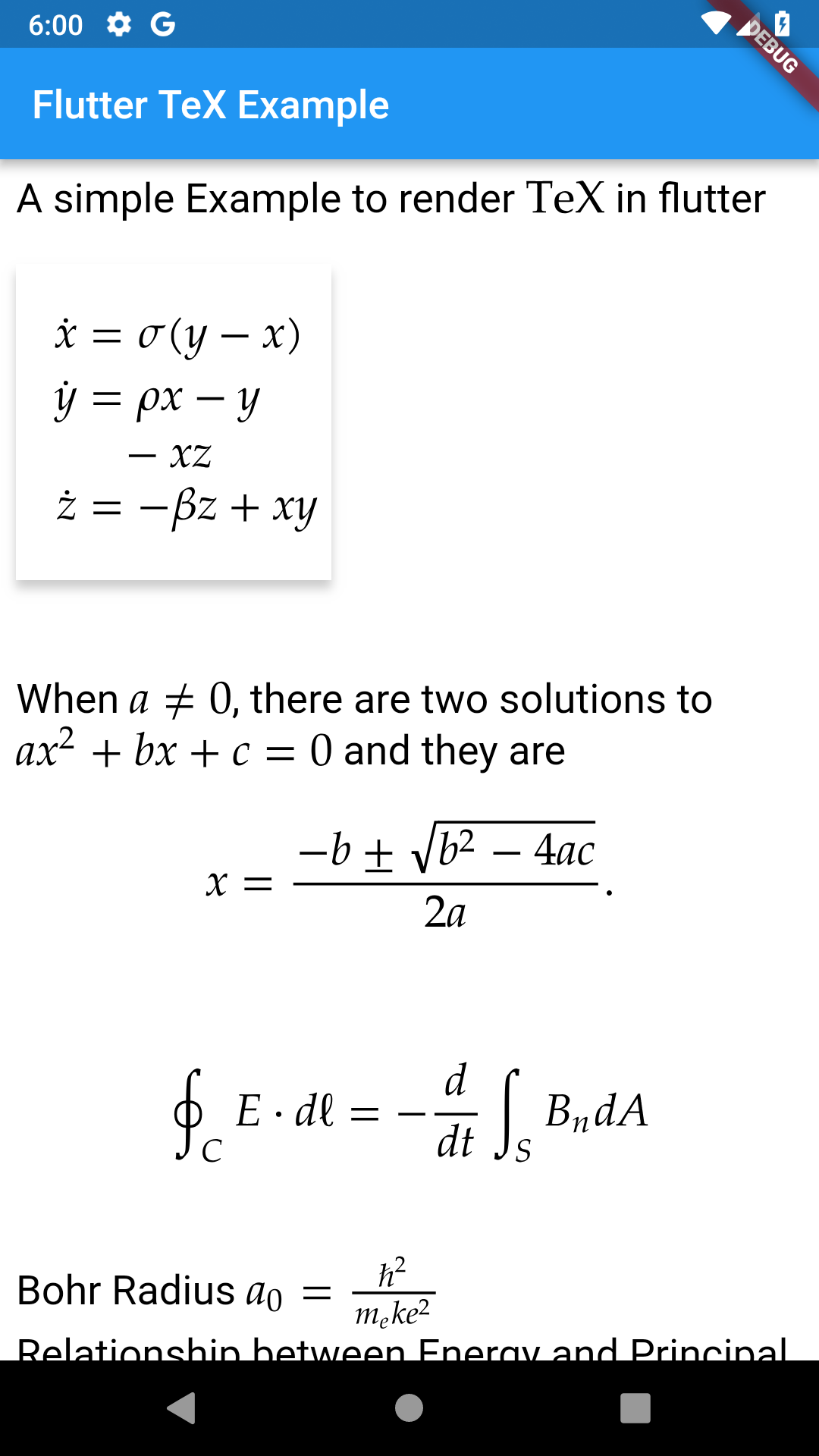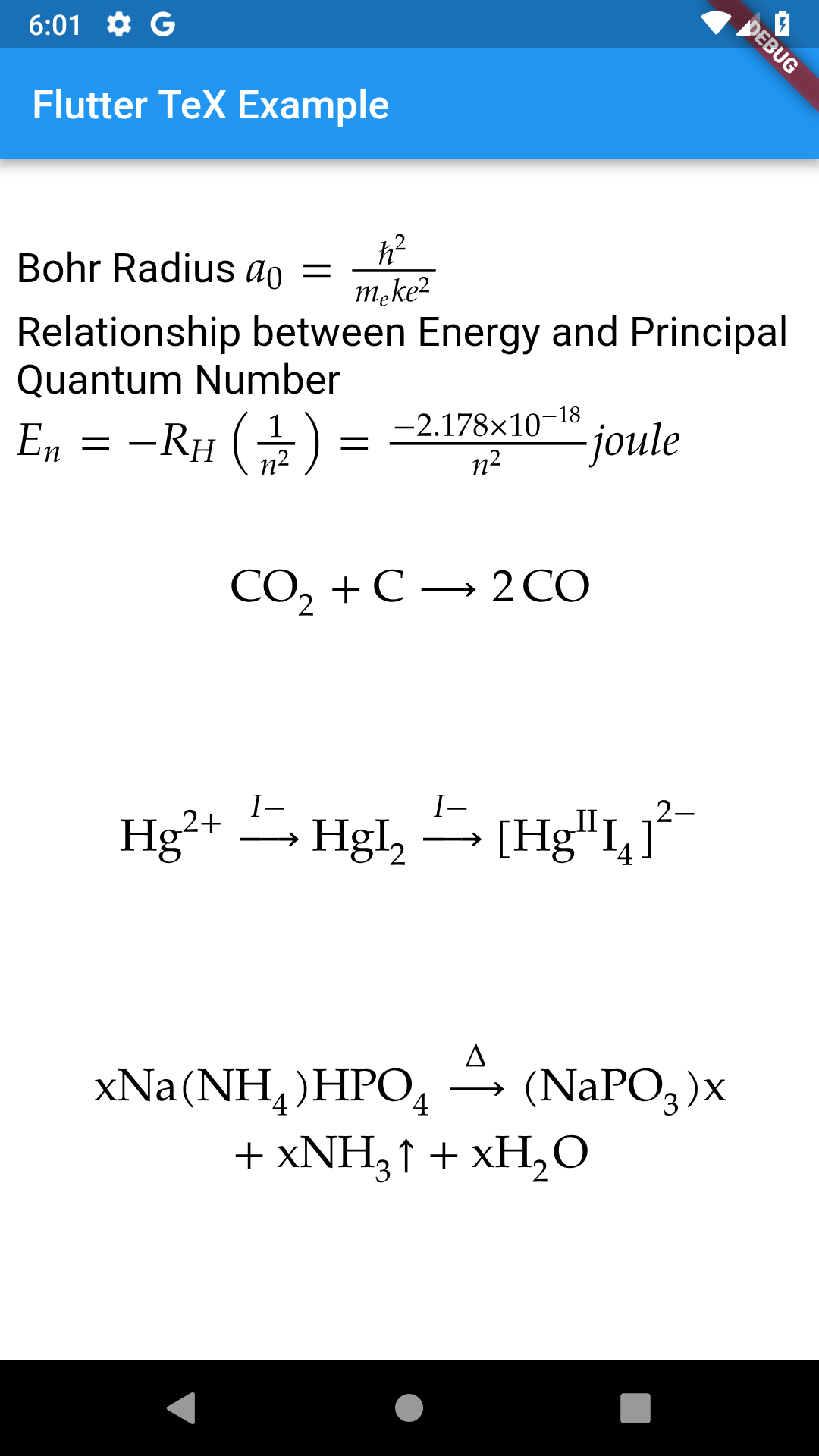/ Calculator

# A Flutter Package to render Mathematics and Chemistry Equations based on LaTeX## Flutter TeX

A Flutter Package to render so many types of equations based on LaTeX, most commonly used are as followings:

• Mathematics / Maths Equations (Algebra, Calculus, Geometry, Geometry etc...)

• Physics Equations

• Signal Processing Equations

• Chemistry Equations

• Statistics / Stats Equations

• It also includes full HTML with JavaScript support.

Rendering of equations depends on mini-mathjax a simplified version of MathJax a JavaScript library.

This package mainly depends on webview_flutter plugin.

Only Tested on Android not on iOS because I don't own a Mac.

## Use this package as a library in your flutter Application

dependencies:
flutter_tex: ^0.0.19


2: You can install packages from the command line:

\$ flutter packages get


3: Now in your Dart code, you can use:

import 'package:flutter_tex/flutter_tex.dart';


4: Make sure to add this line android:usesCleartextTraffic="true" in your <project-directory>/android/app/src/main/AndroidManifest.xml under application like this.

<application
android:usesCleartextTraffic="true">
</application>


For iOS add following code in your <project-directory>/ios/Runner/Info.plist


<key>NSAppTransportSecurity</key>

<dict>
</dict>

<key>io.flutter.embedded_views_preview</key> <true/>


## Example

import 'package:flutter/material.dart';
import 'package:flutter_tex/flutter_tex.dart';

main() async {
runApp(FlutterTeX());
}

class FlutterTeX extends StatefulWidget {
@override
_FlutterTeXState createState() => _FlutterTeXState();
}

class _FlutterTeXState extends State<FlutterTeX> {
String teX = Uri.encodeComponent(r"""
<p>
A simple Example to render $$\rm\\TeX$$ in flutter<br>

<style>
.card {
box-shadow: 0 4px 8px 0 rgba(0, 0, 0, 0.2);
transition: 0.3s;
width: 40%;
}

.card:hover {
box-shadow: 0 8px 16px 0 rgba(0, 0, 0, 0.2);
}

.container {

</style>
<div class="card">
<div class="container">
<p>
\begin{align}
\dot{x} & = \sigma(y-x) \\
\dot{y} & = \rho x - y - xz \\
\dot{z} & = -\beta z + xy
\end{align}
</p>
</div>
</div>

<br>
<br>

When $$a \ne 0$$, there are two solutions to $$ax^2 + bx + c = 0$$ and they are

$$x = {-b \pm \sqrt{b^2-4ac} \over 2a}.$$<br>

$$\oint_C {E \cdot d\ell = - \frac{d}{{dt}}} \int_S {B_n dA}$$<br>

$$a_0 = \frac{{\hbar ^2 }}{{m_e ke^2 }}$$<br>

Relationship between Energy and Principal Quantum Number

$$E_n = - R_H \left( {\frac{1}{{n^2 }}} \right) = \frac{{ - 2.178 \times 10^{ - 18} }}{{n^2 }}joule$$<br><br>

$$\ce{CO2 + C -> 2 CO}$$ <br><br>

$$\ce{Hg^2+ ->[I-] HgI2 ->[I-] [Hg^{II}I4]^2-}$$ <br><br>

$$\ce{x Na(NH4)HPO4 ->[\Delta] (NaPO3)_x + x NH3 ^ + x H2O}$$ <br><br>

</p>
""");

@override
Widget build(BuildContext context) {
return MaterialApp(
home: Scaffold(
appBar: AppBar(
title: Text("Flutter TeX Example"),
),
body: TeXView(
teXHTML: teX,
),
),
);
}
}


## Screenshots## GitHub#### Flutter Awesome

An awesome list that curates the best Flutter libraries and tools.

Latest
Tag